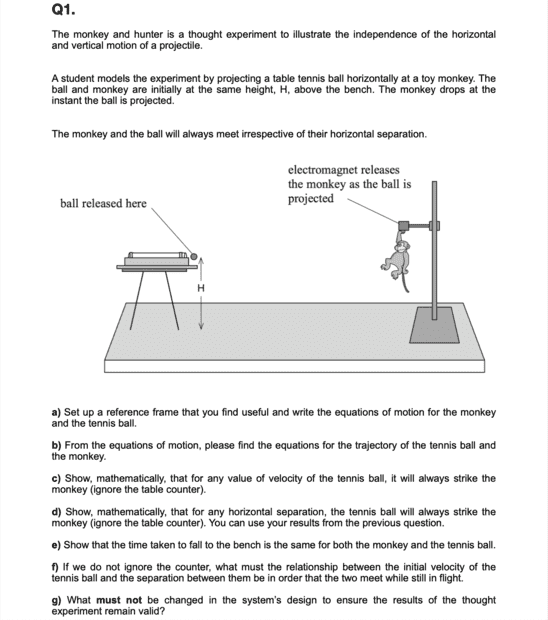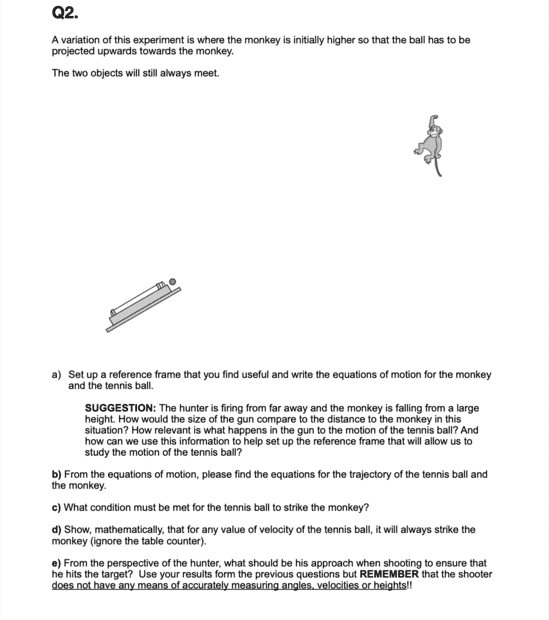# Kinematics in Two Dimensions (Monkey and Hunter Thought Experiment)

griffin_neal
Poster warned for not providing an attempt at a solution
Homework Statement:
This week, I was assigned this assignment, which is really giving me a tough time. It involves the classic monkey and hunter thought experiment. I am at a total loss at how to even approach this question. Here is what the question asks:

"
The monkey and hunter is a thought experiment to illustrate the independence of the horizontal and vertical motion of a projectile.
A student models the experiment by projecting a table tennis ball horizontally at a toy monkey.
The ball and monkey are initially at the same height, H, above the bench. The monkey drops the instant the ball is projected.
The monkey and the ball will always meet, irrespective of their horizontal separation.

a) Set up a reference frame that you find useful and write the equations of motion for the monkey
and the tennis ball.
b) From the equations of motion, please find the equations for the trajectory of the tennis ball and the monkey.
c) Show, mathematically, that for any value of velocity of the tennis ball, it will always strike the
monkey (ignore the table counter)
d) Show, mathematically, that for any horizontal separation, the tennis ball will always strike the
monkey (ignore the table counter). You can use your results from the previous question.
e) Show that the time taken to fall to the bench is the same for both the monkey and the tennis ball.
f) If we do not ignore the counter, what must the relationship between the initial velocity of the
tennis ball and the separation between them be in order that the two meet while still in flight?
g) What must not be changed in the system's design to ensure the results of the thought
experiment remain valid?
"
Relevant Equations:
All SUVAT equations of motion:

s = ([u + v]t)/2
v = u + at
s = ut + 1/2at^2
v2 =u^2 + 2asHomework Helper
Gold Member
2022 Award
I am at a total loss at how to even approach this question.
That won't do. Per forum rules, you must show some attempt.
There are many parts. You must at least have some thoughts on some of them.

•